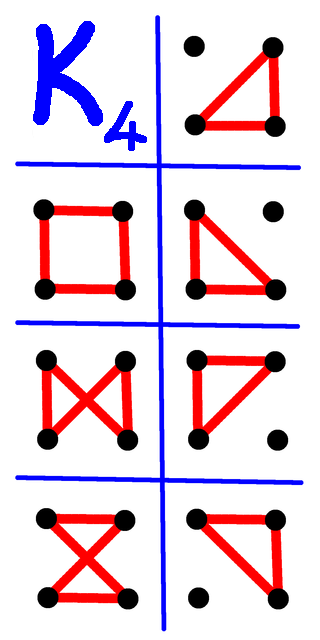# Vertices Required For CyclesSubscribe!My latest posts can be found here:
Previous blog posts:

## Minimal graphs with exactly C cycles ...

Back in late August (the 26th to be precise) Chris Purcell (@ccppurcell@mathstodon.xyz) posted the following:

 Here's a sequence that doesn't appear to be in the OEIS (unless I erred). Let $a_n$ be the size of the smallest graph with exactly n cycles. The first few terms are: 3, 5, 4, 6, ...

The first few values are easy enough to compute by hand, but it gets quite tricky quite quickly. How can one construct a (simple undirected) graph with exactly 5 cycles?

## Exploring the problem

We start by taking a few small graphs and counting the cycles. For a triangle we have exactly one cycle. For a square we also have exactly one cycle, but we have a smaller example for that, so we are not interested in the square.

A square with one diagonal has two cycles of length three, and one cycle of length four, giving 3 cycles in total.Cycles in $K_4$
A square with both diagonals is the complete graph on four vertices. You might think that it has four cycles of length three (and you'd be right) and one cycle of length four, being the circuit when you draw it as a square, but that is not the entire story. We also have the "bow tie" and the "hour-glass" cycles, so $K_4$ has a total of seven cycles.

## Constructing an example

To construct a graph with exactly $k$ cycles we can take $k$ triangles with a shared vertex. That gives the desired $k$ cycles with $2k+1$ vertices, but perhaps we can do better.

To get 5 cycles, for example, we could start with a square with one diagonal, which has three cycles. Then we add two extra triangles, again sharing a vertex. That gives us the desired 5 cycles using only 8 vertices instead of 9. So we now know that $V(5) <= 8$.

Can we do better? In fact, no, but proving so seems to be a trifle awkward. So we have a few questions:

• How can we construct graphs with exactly C cycles?
• How can we know that it is a minimal example?
• How quickly does the function grow?

We have a few things. If we know that we can construct exactly C cycles using V vertices, then we can construct exactly C+1 cycles using V+2 cycles.

But in general we can accomplish many cycles with many fewer vertices. User 0xDE (@11011110@mathstodon.xyz) made the following observation:

 Replacing each edge of an $n$-gon by a triangle gives number of cycles $2^n+n$ (two choices at each edge if you go all the way around, plus the triangles), with $O(n)$ vertices and edges. Now for any build a graph whose biconnected components look like this, choosing greedily at each step the biggest polygon you can use. Result: exactly $n$ cycles using $O(\log n)$ vertices and edges. Probably with care you can reduce vertices to $O(\log n/\log\log n).$

So we can get many, many cycles with not many vertices, but we won't necessarily get all possible number of cycles. With four vertices we can get 0, 1, 3, or 7 cycles, but not 2, 5, or 6. Even so, the required number of vertices certainly won't grow quickly. The complete graph on $k$ vertices has more than $(k-1)!$ cycles, and that's a lot.

## What I did ...

 I used geng from the nauty suite to generate the graphs, and then wrote a program to count the cycles in each.
To investigate, I wrote a program to compute the number of cycles in all graph up to 10 vertices. The first value that is unknown (to me) is 18902. I know that there is a graph of 10 vertices with exactly 18901 cycles, and another with exactly 18903 cycles. So the minimum number of vertices required for a graph to have exactly 18902 cycles is either 11 or 12, but I don't know which.

The next step with my existing program would be to compute the number of cycles for all 1 billion connected non-isomorphic graphs on 11 vertices, but I estimate that would take around a week of runtime, so it's time to stop and wonder what the next interesting question might be.

I'm happy to provide my code to anyone sufficiently interested, or to describe the process and algorithms in some detail in case people would like to implement them independently, but to be honest, they're none of interesting, clever, or innovative.

## The OEIS ...

The sequence is now in the OEIS:

I have the first 18900 values in case anyone wants them.

I've had some feedback on this post, which has been absolutely brilliant, but I'm keen to get more. Did you find this easy to read? Was there something that confused you? Do you want to know more about some part of it?

The comment box can be completely anonymous, or you can put your email in the appropriate place if you'd like a reply. Alternatively, ping me on Mathstodon or Twitter. I know I don't always hit the mark for level of detail, so please, do let me know.

 <<<< Prev <<<< Reflex Actions : >>>> Next >>>> Pending ...You can follow me on Mathstodon.

 Of course, you can alsofollow me on twitter:## Send us a comment ...

 You can send us a message here. It doesn't get published, it just sends us an email, and is an easy way to ask any questions, or make any comments, without having to send a separate email. So just fill in the boxes and then

 Your name : Email : Message :

# ContentsSuggest a change ( <-- What does this mean?) / Send me email